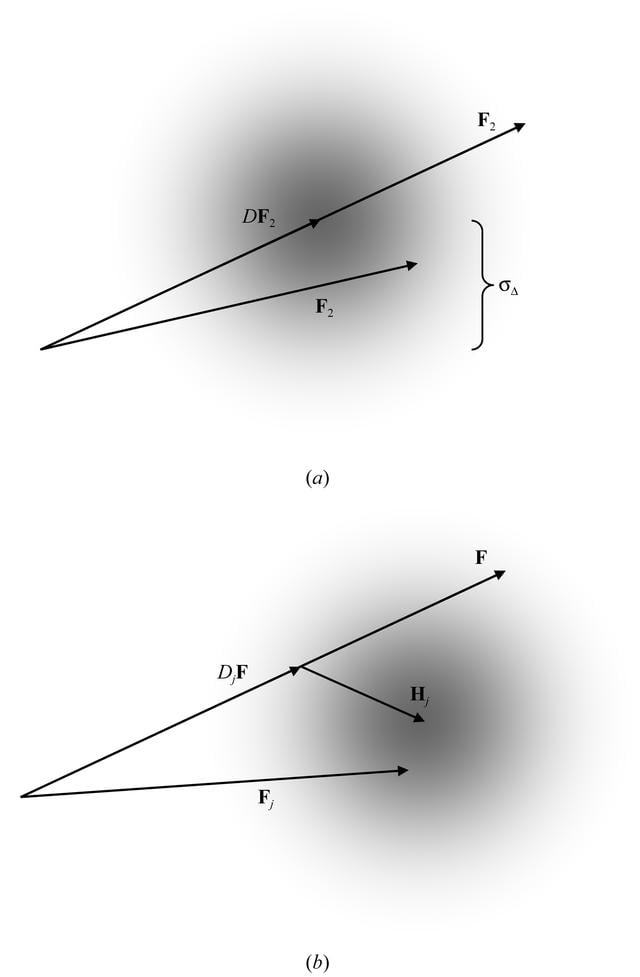disable zoom     view article Figure 1 Schematic representation of conditional probability distributions of structure factors. (a) Probability distribution (represented by grey shading) of structure factor F1 given a related structure factor F2. The distribution, given in (1), is centred on DF2, where D represents the fraction of F2 that is correlated with F1. The width of the distribution is described by the parameter σΔ. (b) Probability distribution (represented by grey shading) of Fj (structure factor for derivative j) given an assumed true native structure factor F and the structure-factor contribution Hj computed from a heavy-atom model. The distribution, given in (5), is centered on DjF + Hj. The width of the distribution includes contributions from lack of isomorphism, errors in the heavy-atom model and measurement error.BIOLOGICALCRYSTALLOGRAPHY
ISSN: 1399-0047
Volume 59| Part 11| October 2003| Pages 1891-1902